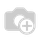# Remaining leave shouldn't be carried over to the next year, can it start over?

In our company, the leave allocation are the same for every employee. But the remaining leave can't be carried over to next year. If the employee takes all the leave allowed by the company, it is fine. The problem is when there are remaining leaves, I want to reset the remaining legal leave to zero so that I do the allocation only once and for all at the beginning of the year.

In odoo, it is not easy to the understand the leave analysis. Because when new allocation is made, it will be added to the remaining leave which is the not the case that we want. We want the report to show the new allocation for particular employee, not to add the past and the new together. I want to start over, so how can I achieve this kind of scenario in ODOO?Hello,

I'm faced with a similar scenario. But I need to move staff leave to the next year.

So, I wrote a scheduled action for this situation based on start dates and ages. But I'm not sure of the accuracy, maybe it can give you an idea.

I created two fields in hr.employee object :

class HrEmployee(models.Model):

_inherit = 'hr.employee'

age = fields.Char(string='Yaş')

annual_leave_calc_number = fields.Integer(string="Annual Leave Calculation Number")

annual_leave_calc_date = fields.Datetime(string="Annual Leave Calculation Date")

After , method for ir_cron

@api.model

def _cron_employee_remaining_leaves(self):

for employee in self.search([('active', '=', True)]):

_emp_leave_right = 0

_emp_age = employee.age

_emp_date_job_start = datetime.strptime(employee.ise_giris_tarihi, '%Y-%m-%d').date()

_current_date = datetime.now().date()

if int(_emp_age) >= 15:

.... (some calculations )

#After:

temp_start_date = _emp_date_job_start

if employee.annual_leave_calculation_number > 0:

temp_start_date = employee.annual_leave_calculation_date

employee.remaining_leaves += _emp_leave_right

employee.annual_leave_calc_date = _current_date

employee.annual_leave_calc_number += 1

else:

_logger.info('15 yaşından küçük personel çalıştıramazsınız ve izin hakkı yoktur.')

# This method for getting next year or years from the starting date